Next: The Clean and Dirty Limits Up: Magnetic Properties of Conventional Superconductors Previous: The Coherence Length (Pippard's Equation)

## Ginzburg-Landau Theory

In 1950, Ginzburg and Landau introduced the so-called superconducting order parameter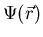to describe the nonlocality of the superconducting properties.can be thought of as a measure of the order in the superconducting state at position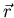below Tc. It has the properties that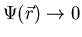as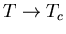, and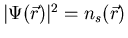(i.e. the local density of superconducting electrons). At the time Ginzburg-Landau (GL) theory was developed, the nature of the superconducting carriers was yet to be determined. Interpreting m* as the effective mass and q as the charge of the fundamental superconducting particles (whatever they may be), they derived the penetration depth as: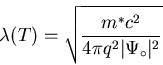(6)

where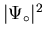is the value of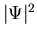deep inside the superconductor (i.e. its equilibrium value). The coherence length defined in the GL-formalism is: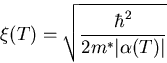(7)

where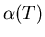is a temperature-dependent coefficient in the series expansion of the free energy (see ). As written, (2.6) and (2.7) suggest that the penetration depth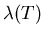and the coherence length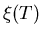are temperature dependent quantities. Eq. (2.7) is closely related to the Pippard coherence length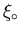defined in Eq. (2.4). In GL-theory, the coherence lengthis the characteristic length for variations in. One noteable difference is that Pippard's coherence lengthis independent of temperature. In fact in the dirty limit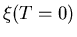reduces to Eq. (2.4). Near the transition temperature Tc bothandvary as (1-T/Tc)-1/2, prompting the introduction of the Ginzburg-Landau parameter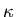, where: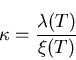(8)

The variation ofandwith temperture near Tccomes from an expansion of the free energy density to first-order in the field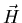. It follows thatin (2.8) is temperature independent to this order. An exact calculation from microscopic theory gives a weak temperature dependence for, withincreasing for decreasing temperature T. Through energy considerations, Ginzburg and Landau characterized a type-I superconductor as one in which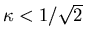. For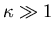, GL theory reduces to the London model.Next: The Clean and Dirty Limits Up: Magnetic Properties of Conventional Superconductors Previous: The Coherence Length (Pippard's Equation)
Jess H. Brewer
2001-09-28